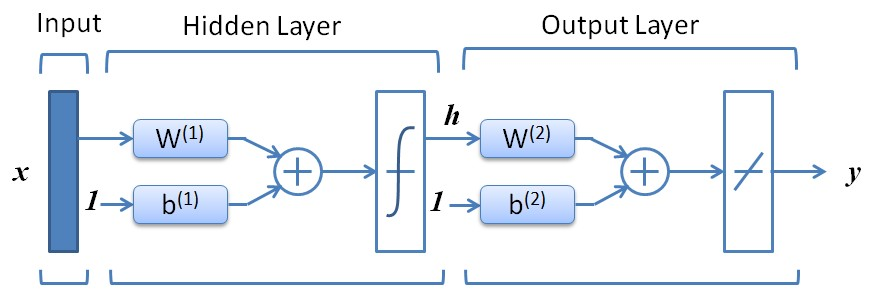## 1.概述

BP（Back Propagation）神经网络是1986年由Rumelhart和McCelland为首的科研小组提出，参见他们发表在Nature上的论文 Learning representations by back-propagating errors 值得一提的是，该文的第三作者Geoffrey E. Hinton就是在深度学习邻域率先取得突破的神犇。

BP神经网络是一种按误差逆传播算法训练的多层前馈网络，是目前应用最广泛的神经网络模型之一。BP网络能学习和存贮大量的 输入-输出模式映射关系，而无需事前揭示描述这种映射关系的数学方程。它的学习规则是使用最速下降法，通过反向传播来不断 调整网络的权值和阈值，使网络的误差平方和最小。

## 2.BP网络模型BP神经网络与其他神经网络模型类似，不同的是，BP神经元的传输函数为非线性函数(而在感知机中为阶跃函数，在线性神经网络中为线性函数)，最常用的 是log-sigmoid函数或tan-sigmoid函数。BP神经网络(BPNN)一般为多层神经网络，图1中所示的BP神经网络的隐层的传输函数即为非线性函数，隐层可以有多层， 而输出层的传输函数为线性函数，当然也可以是非线性函数，只不过线性函数的输出结果取值范围较大，而非线性函数则限制在较小范围（如logsig函数输出 取值在(0,1)区间）。图1所示的神经网络的输入输出关系如下：
1)输入层与隐层的关系：

$\boldsymbol{h} = \mathit{f_{1}} (\boldsymbol{W^{(1)}x}+\boldsymbol{b^{(1)}})$.

2)隐层与输出层的关系：
$\boldsymbol{y} = \mathit{f_{2}} (\boldsymbol{W^{(2)}h}+\boldsymbol{b^{(2)}})$.

## 3.BP网络的学习方法

$\boldsymbol{E(W,B)} = \frac{1}{2}\sum_{k=1}^{n}(t_{k} - a2_{k})^{2}$.
BP算法的目标是使得实际输出approximate期望输出，即使得训练误差最小化。BP算法利用梯度下降(Gradient Descent)法来求权值的变化及 误差的反向传播。对于图1中的BP神经网络，我们首先计算输出层的权值的变化量，从第$i$个输入到第$k$个输出的权值改变为：
$\Delta w2_{ki} = - \eta \frac{\partial E}{\partial w2_{ki}} \\ = - \eta \frac{\partial E}{\partial a2_{k}} \frac{\partial a2_{k}}{\partial w2_{ki}} \\ = \eta (t_{k}-a2_{k})f_{2}'a1_{i} = \eta \delta_{ki}a1_{i}$.

$\Delta b2_{ki} = - \eta \frac{\partial E}{\partial b2_{ki}} = - \eta \frac{\partial E}{\partial a2_{k}} \frac{\partial a2_{k}}{\partial b2_{ki}} = \eta (t_{k}-a2_{k})f_{2}' = \eta \delta_{ki}$.

$\Delta w1_{ij} = - \eta \frac{\partial E}{\partial w1_{ij}} = - \eta \frac{\partial E}{\partial a2_{k}} \frac{\partial a2_{k}}{\partial a1_{i}} \frac{\partial a1_{i}}{\partial w1_{ij}} = \eta \sum_{k=1}^{n}(t_{k}-a2_{k})f_{2}'w2_{ki}f_{1}'p_{j} = \eta \delta_{ij}p_{j}$.

## 4.BP网络的设计

1.网络的层数

2.隐层神经元的个数

（1）神经元数太少时，网络不能很好的学习，训练迭代的次数也比较多，训练精度也不高。
（2）神经元数太多时，网络的功能越强大，精确度也更高，训练迭代的次数也大，可能会出现过拟合(over fitting)现象。

3.初始权值的选取

4.学习速率

5.期望误差的选取

## 5.BP网络的局限性

BP网络具有以下的几个问题：
(1)需要较长的训练时间：这主要是由于学习速率太小所造成的，可采用变化的或自适应的学习速率来加以改进。
(2)完全不能训练：这主要表现在网络的麻痹上，通常为了避免这种情况的产生，一是选取较小的初始权值，而是采用较小的学习速率。
(3)局部最小值：这里采用的梯度下降法可能收敛到局部最小值，采用多层网络或较多的神经元，有可能得到更好的结果。

## 6.BP网络的改进

BP算法改进的主要目标是加快训练速度，避免陷入局部极小值等，常见的改进方法有带动量因子算法、自适应学习速率、变化的学习速率以及作用函数后缩法等。 动量因子法的基本思想是在反向传播的基础上，在每一个权值的变化上加上一项正比于前次权值变化的值，并根据反向传播法来产生新的权值变化。而自适应学习 速率的方法则是针对一些特定的问题的。改变学习速率的方法的原则是，若连续几次迭代中，若目标函数对某个权倒数的符号相同，则这个权的学习速率增加， 反之若符号相反则减小它的学习速率。而作用函数后缩法则是将作用函数进行平移，即加上一个常数。

## 参考文献

An Introduction to Back-Propagation Neural Networks: http://www.seattlerobotics.org/encoder/nov98/neural.html
Wiki - Backpropagation: http://en.wikipedia.org/wiki/Backpropagation
Chapter 7 The backpropagation algorithm of Neural Networks - A Systematic Introduction by Raúl Rojas: http://page.mi.fu-berlin.de/rojas/neural/chapter/K7.pdf
Back-propagation Neural Net - C++ 实现: http://www.codeproject.com/Articles/13582/Back-propagation-Neural-Net
《Visual C++数字图像模式识别技术及工程实践》(第3章)，求实科技 张宏林
《Matlab神经网络设置及应用》(第5章)，周品，清华大学出版社